View Part: 1 2 3 4 5 6 7 8 9 10 11 12

PART 3 - NAZCA

The glyphs and lines at Nazca are oriented along the alignment of ancient sites. The image below of the glyphs at Nazca with a compass bearing, is available on the internet, but it is usually oriented away from the cardinal points so that the figures are roughly horizontal and vertical. Rotating this image so that the north-south axis is vertical, aligns most of the figures and geometric drawings with the alignment of ancient sites as it crosses Nazca.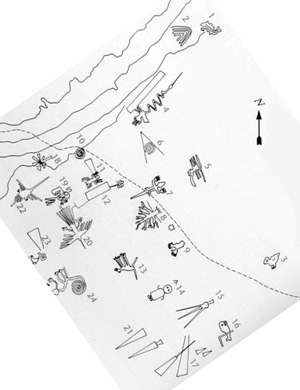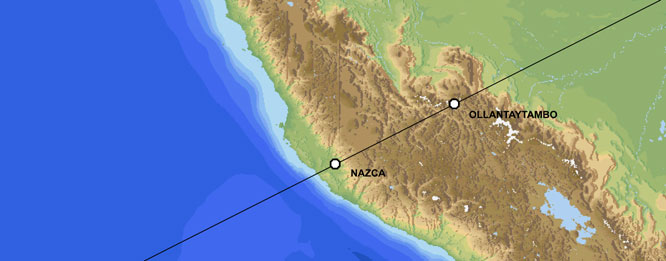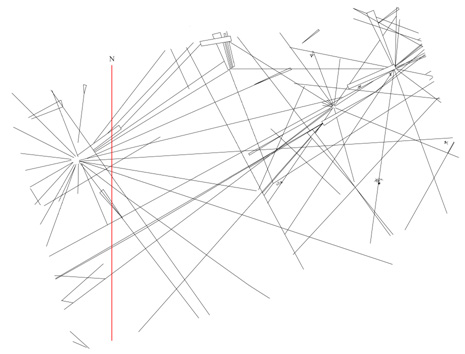The illustration of the Nazca lines above has also been rotated so that the north-south axis is vertical, and shows the primary orientation of the lines is from southwest to northeast, matching the great circle alignment of ancient sites as it crosses Nazca.

The distance from the Nazca lines to Giza is 7692 miles. The distance from the axis point in southeastern Alaska to Giza and to Nazca is 6218 miles, forming an isosceles terrestrial triangle with a baselength of 7692 miles and sidelengths of 6218 miles.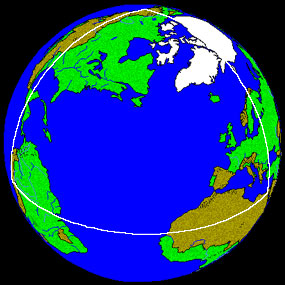The 3D global image above is centered in the middle of the terrestrial triangle. As a result, all three of the great circle segments are curved. The equal azimuthal projection below is centered on the axis point. As a result, the great circle segments from the axis point to Giza and to Nazca are straight lines. 7692 miles is 30.9% of the circumference of the great circle (7692/24,892 = .309) or 111.245° along the great circle (.309 x 360 = 111.245 ).

The azimuth of the great circle segment from the axis point to Giza is 9° and the azimuth of the great circle segment from the axis point to Nazca is 120°, showing that the angle at the axis point is also 111°.

The distance from the axis point to Giza and to Nazca is one quarter of the circumference of the earth, or 90°. Since the great circle segments from the axis point are perpendicular to the great circle itself, the angles at Giza and Nazca are also 90°.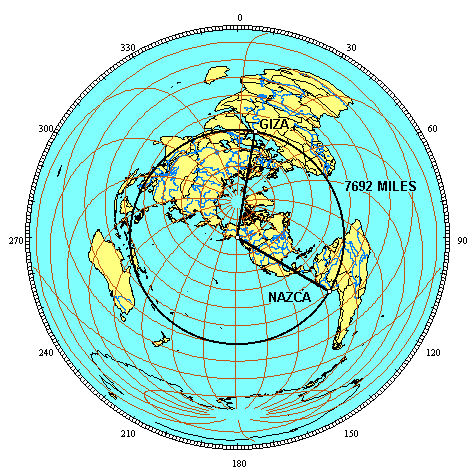A simple spherical trigonometry formula converts the 90° - 90° - 111° spherical triangle to an equivalent flat surface triangle. The angle opposite the baselength is divided by two (111.235°/2 = 55.6225°), and then divided by the angle adjacent to the baselength (55.6225°/90° = .618). The φ reciprocal is .618 (1/φ). Applying the inverted cosine function to .618 gives 51.83° for the flat surface equivalents of the 90° spherical angles at Nazca and Giza.

These are the same as the angular dimensions of the great pyramid. Alternatively, the two great circle segments from the axis point to Nazca and from the axis point to Giza are each 25% of the circumference of the earth while the great circle segment from Nazca to Giza is 30.9% of the circumference of the earth.

The two great circle segments from the axis point to Nazca and to Giza add up to 50% of the circumference of the earth and 50/30.9 = 1.618, also demonstrating that these three great circle segments form a triangle equivalent to the crossection of the great pyramid, with the same φ proportion: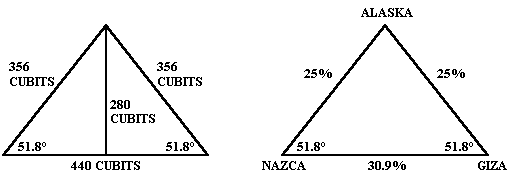This relationship may also be shown using the distance between the three points in miles. One quarter of the circumference of the earth (along the great circle alignment) is 6223 miles (24,892/4 = 6223). The two sides of the terrestrial triangle are 6223 miles long, for a combined length of 12,446 miles. The distance from Giza to Nazca is 7692 miles and 12,446/7692 = 1.618. The above calculation is based on a round earth assumption. Although the great circle segments from the axis point to the great circle are 90° or one quarter of the circumference of the great circles from the axis points, the circumference of the great circles that cross over the axis point are actually a bit shorter than the circumference of the main great circle because the axis point is closer to the pole than the primary great circle that crosses over Giza and Nazca.

The mean distance from the axis point to the great circle is 6218 miles, five miles less than one quarter of the circumference of the primary great circle, just as the distance from the north and south poles to the equator is 6215 miles even though one quarter of the equatorial circumference of the earth is 6225 miles (24,901/4 = 6225).

Given side lengths of 6218 miles from the axis point to Giza and Nazca, the distance from Giza to Nazca would have to be 7686 miles for the φ ratio to be exact, based on an ellipsoidal earth model. The main group of lines and figures at Nazca that is included as a UNESCO world heritage site is approximately 25 miles E-W and 4 miles N-S.

The distance of 7692 miles from Giza along the great circle and the distance of 7686 miles from Giza along the great circle both fall within the main part of the Nazca lines and figures that are designated by UNESCO for protection as a world heritage site, so in the case of Nazca the φ relationship is present with either a round earth assumption or an ellipsoidal model.